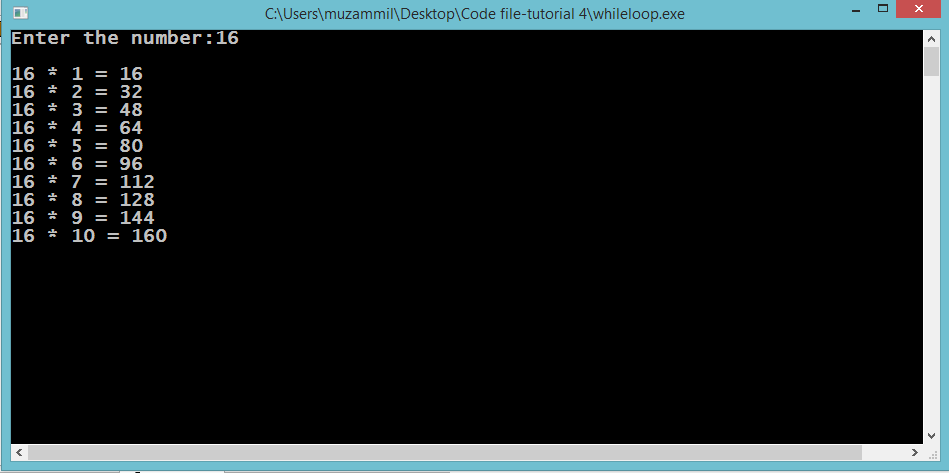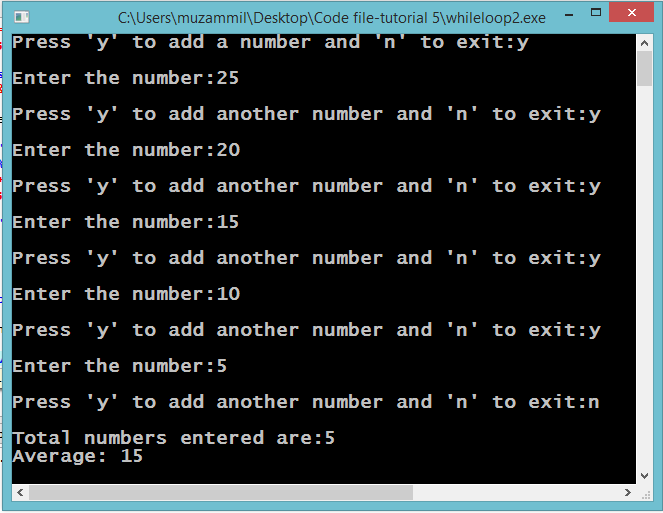# While Loops

## While loops

In this part you will learn: 1. C syntax 2. While loops 3. More about Conditional Statements In this tutorial I will teach you about While loops. We will see two programs that use while loops. The first one will calculate the table of the number that user enters and the second one will calculate the average of a sum of numbers. Calculating Table Basic Step: Open Dev C++ then File > new > source file and start writing the code below.
`#include<stdio.h>#include<conio.h>`
These two are the most common and basic header files. The first one stdio.h is used to grant us most of the basic functions of C like Input and Output functions. The second one conio.h provides us functions like getch() which is used to pause our screen until we press a button.
`int main(){	int n,p; 	printf("Enter the number:");	scanf("%d",&n); 	int i=1;	while(i<=10)	{		p=n*i;		printf("\n%d * %d = %d",n,i,p);		i++;	} 	getch();}`
In this program we will print the table of a number that user enters using a while loop. A while loop is just like a for loop. Like for loop it also has three statements but they are optional. 1. Initialization 2. Condition 3. Increment The difference between a for loop and while loop comes in the places where these statements are written. For a while loop we write the initializing statement outside the while loop. In this program you can see before the start of while loop we have initialized the counter variable ‘i’. Then in the brackets of the while loop we write the condition. The condition we have in this program is till our counter variable is less than or equal to 10, our loop should run. Then comes the body of the loop. In the body of the loop we are multiplying the number ‘n’ which we took from the user with our counter variable and storing it in another variable ‘p’. then we are printing the value of ‘p’. After that we increment in the counter. Then when the counter is greater than 10 the loop breaks and we come to the getch() function which pauses the program. Execute > compile then Execute > run OutputCalculating Average Basic Step: Open Dev C++ then File > new > source file and start writing the code below.
`#include<stdio.h>#include<conio.h>`
These two are the most common and basic header files. The first one stdio.h is used to grant us most of the basic functions of C like Input and Output functions. The second one conio.h provides us functions like getch() which is used to pause our screen until we press a button.
`int main(){	int n,count=0,sum=0,avg=0;	char choice; 	printf("Press 'y' to add a number and 'n' to exit:");	scanf("%c",&choice); 	while(choice=='y')	{		printf("\nEnter the number:");		scanf("%d",&n);		sum=sum+n;		count++; 		printf("\nPress 'y' to add another number and 'n' to exit:");		scanf(" %c",&choice); 	} 	printf("\nTotal numbers entered are:%d",count);	if(count>0)	avg=sum/count; 	printf("\nAverage: %d",avg); 	getch();  }`
In this program we our calculating the average of sum of multiple numbers entered by the user at run time. We are doing this by asking the user if he wants to enter a number or not. We take input from the user in a character variable which is either ‘y’ or ‘n’. If the user enters ‘y’ we enter the loop as the entering condition of our loop is when the user enters ‘y’ the program should enter the while loop. Note that in this case we have no initializing statement. This is a difference between a for loop and while loop. You cannot miss initializing in for loop but you can write a while loop without any initialization. The only this necessary for the while loop is the condition. The increment statement can also be neglected depending upon the program you are writing. Now after entering the loop we take input the number from the user and add it to an accumulator variable ‘sum’. This variable contains the sum of all the numbers entered by the user. Then afterwards we increment a counter variable which keeps the track of total numbers entered by the user. And then there is the last statement where we are taking input from the user that either he wants to enter a new number or he wants to exit the loop so that he can see the average. Outside the body of the loop we are printing the total numbers that the user entered. Than we place a check that if the count is greater than 0 then the program should calculate average. This is because if the count is 0 and then the sum will be 0 too. And when we divide sum of numbers by total numbers which will be both 0 so it will become infinity and cause an error. The at last after printing the average there is getch() function. And afterward the program ends. Execute > compile then Execute > run Output